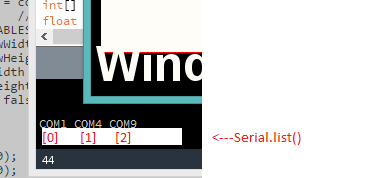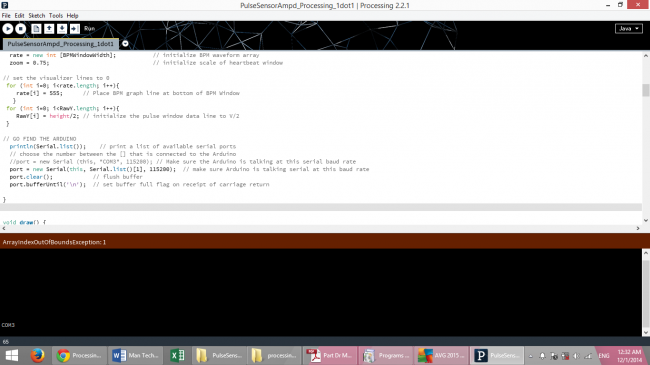#### Howdy, Stranger!

We are about to switch to a new forum software. Until then we have removed the registration on this forum.

# Processing Code to Display Amped Visualizer

edited April 2015 in Arduino

Hello guys, I need a helping hand here. I am new to this processing software and i obtained a processing code along with my brand new Pulse Sensor. I successfully call out the visualizer ( printed screen below ), but there was no reading on the graph, it just drop to zero although my pulse sensor is working perfectly with Arduino Uno. Please help me....thanks anywayHere is my code:

``````/**
THIS PROGRAM WORKS WITH PulseSensorAmped_Arduino-xx ARDUINO CODE
THE PULSE DATA WINDOW IS SCALEABLE WITH SCROLLBAR AT BOTTOM OF SCREEN
PRESS 'S' OR 's' KEY TO SAVE A PICTURE OF THE SCREEN IN SKETCH FOLDER (.jpg)
MADE BY JOEL MURPHY AUGUST, 2012
*/

import processing.serial.*;
PFont font;
Scrollbar scaleBar;

Serial port;

int Sensor;      // HOLDS PULSE SENSOR DATA FROM ARDUINO
int IBI;         // HOLDS TIME BETWEN HEARTBEATS FROM ARDUINO
int BPM;         // HOLDS HEART RATE VALUE FROM ARDUINO
int[] RawY;      // HOLDS HEARTBEAT WAVEFORM DATA BEFORE SCALING
int[] ScaledY;   // USED TO POSITION SCALED HEARTBEAT WAVEFORM
int[] rate;      // USED TO POSITION BPM DATA WAVEFORM
float zoom;      // USED WHEN SCALING PULSE WAVEFORM TO PULSE WINDOW
float offset;    // USED WHEN SCALING PULSE WAVEFORM TO PULSE WINDOW
color eggshell = color(255, 253, 248);
int heart = 0;   // This variable times the heart image 'pulse' on screen
//  THESE VARIABLES DETERMINE THE SIZE OF THE DATA WINDOWS
int PulseWindowWidth = 490;
int PulseWindowHeight = 512;
int BPMWindowWidth = 180;
int BPMWindowHeight = 340;
boolean beat = false;    // set when a heart beat is detected, then cleared when the BPM graph is advanced

void setup() {
size(700, 600);  // Stage size
frameRate(100);
textFont(font);
textAlign(CENTER);
rectMode(CENTER);
ellipseMode(CENTER);
// Scrollbar constructor inputs: x,y,width,height,minVal,maxVal
scaleBar = new Scrollbar (400, 575, 180, 12, 0.5, 1.0);  // set parameters for the scale bar
RawY = new int[PulseWindowWidth];          // initialize raw pulse waveform array
ScaledY = new int[PulseWindowWidth];       // initialize scaled pulse waveform array
rate = new int [BPMWindowWidth];           // initialize BPM waveform array
zoom = 0.75;                               // initialize scale of heartbeat window

// set the visualizer lines to 0
for (int i=0; i<rate.length; i++){
rate[i] = 555;      // Place BPM graph line at bottom of BPM Window
}
for (int i=0; i<RawY.length; i++){
RawY[i] = height/2; // initialize the pulse window data line to V/2
}

// GO FIND THE ARDUINO
println(Serial.list());    // print a list of available serial ports
// choose the number between the [] that is connected to the Arduino
port = new Serial(this, Serial.list(), 115200);  // make sure Arduino is talking serial at this baud rate
port.clear();            // flush buffer
port.bufferUntil('\n');  // set buffer full flag on receipt of carriage return
}

void draw() {
background(0);
noStroke();
// DRAW OUT THE PULSE WINDOW AND BPM WINDOW RECTANGLES
fill(eggshell);  // color for the window background
rect(255,height/2,PulseWindowWidth,PulseWindowHeight);
rect(600,385,BPMWindowWidth,BPMWindowHeight);

// DRAW THE PULSE WAVEFORM
// prepare pulse data points
RawY[RawY.length-1] = (1023 - Sensor) - 212;   // place the new raw datapoint at the end of the array
zoom = scaleBar.getPos();                      // get current waveform scale value
offset = map(zoom,0.5,1,150,0);                // calculate the offset needed at this scale
for (int i = 0; i < RawY.length-1; i++) {      // move the pulse waveform by
RawY[i] = RawY[i+1];                         // shifting all raw datapoints one pixel left
float dummy = RawY[i] * zoom + offset;       // adjust the raw data to the selected scale
ScaledY[i] = constrain(int(dummy),44,556);   // transfer the raw data array to the scaled array
}
stroke(250,0,0);                               // red is a good color for the pulse waveform
noFill();
beginShape();                                  // using beginShape() renders fast
for (int x = 1; x < ScaledY.length-1; x++) {
vertex(x+10, ScaledY[x]);                    //draw a line connecting the data points
}
endShape();

// DRAW THE BPM WAVE FORM
// first, shift the BPM waveform over to fit then next data point only when a beat is found
if (beat == true){   // move the heart rate line over one pixel every time the heart beats
beat = false;      // clear beat flag (beat flag waset in serialEvent tab)
for (int i=0; i<rate.length-1; i++){
rate[i] = rate[i+1];                  // shift the bpm Y coordinates over one pixel to the left
}
// then limit and scale the BPM value
BPM = min(BPM,200);                     // limit the highest BPM value to 200
float dummy = map(BPM,0,200,555,215);   // map it to the heart rate window Y
rate[rate.length-1] = int(dummy);       // set the rightmost pixel to the new data point value
}
// GRAPH THE HEART RATE WAVEFORM
stroke(250,0,0);                          // color of heart rate graph
strokeWeight(2);                          // thicker line is easier to read
noFill();
beginShape();
for (int i=0; i < rate.length-1; i++){    // variable 'i' will take the place of pixel x position
vertex(i+510, rate[i]);                 // display history of heart rate datapoints
}
endShape();

// DRAW THE HEART AND MAYBE MAKE IT BEAT
fill(250,0,0);
stroke(250,0,0);
// the 'heart' variable is set in serialEvent when arduino sees a beat happen
heart--;                    // heart is used to time how long the heart graphic swells when your heart beats
heart = max(heart,0);       // don't let the heart variable go into negative numbers
if (heart > 0){             // if a beat happened recently,
strokeWeight(8);          // make the heart big
}
smooth();   // draw the heart with two bezier curves
bezier(width-100,50, width-20,-20, width,140, width-100,150);
bezier(width-100,50, width-190,-20, width-200,140, width-100,150);
strokeWeight(1);          // reset the strokeWeight for next time

// PRINT THE DATA AND VARIABLE VALUES
fill(eggshell);                                       // get ready to print text
text("Pulse Sensor Amped Visualizer 1.1",245,30);     // tell them what you are
text("IBI " + IBI + "mS",600,585);                    // print the time between heartbeats in mS
text(BPM + " BPM",600,200);                           // print the Beats Per Minute
text("Pulse Window Scale " + nf(zoom,1,2), 150, 585); // show the current scale of Pulse Window

//  DO THE SCROLLBAR THINGS
scaleBar.update (mouseX, mouseY);
scaleBar.display();

//
}  //end of draw loop

//.............................................scalebar (name of file)............................................................................................................

/**
THIS SCROLLBAR OBJECT IS BASED ON THE ONE FROM THE BOOK "Processing" by Reas and Fry
*/

class Scrollbar{
int x,y;               // the x and y coordinates
float sw, sh;          // width and height of scrollbar
float pos;             // position of thumb
float posMin, posMax;  // max and min values of thumb
boolean rollover;      // true when the mouse is over
boolean locked;        // true when it's the active scrollbar
float minVal, maxVal;  // min and max values for the thumb

Scrollbar (int xp, int yp, int w, int h, float miv, float mav){ // values passed from the constructor
x = xp;
y = yp;
sw = w;
sh = h;
minVal = miv;
maxVal = mav;
pos = x - sh/2;
posMin = x-sw/2;
posMax = x + sw/2;  // - sh;
}

// updates the 'over' boolean and position of thumb
void update(int mx, int my) {
if (over(mx, my) == true){
rollover = true;            // when the mouse is over the scrollbar, rollover is true
} else {
rollover = false;
}
if (locked == true){
pos = constrain (mx, posMin, posMax);
}
}

// locks the thumb so the mouse can move off and still update
void press(int mx, int my){
if (rollover == true){
locked = true;            // when rollover is true, pressing the mouse button will lock the scrollbar on
}else{
locked = false;
}
}

// resets the scrollbar to neutral
void release(){
locked = false;
}

// returns true if the cursor is over the scrollbar
boolean over(int mx, int my){
if ((mx > x-sw/2) && (mx < x+sw/2) && (my > y-sh/2) && (my < y+sh/2)){
return true;
}else{
return false;
}
}

// draws the scrollbar on the screen
void display (){

noStroke();
fill(255);
rect(x, y, sw, sh);      // create the scrollbar
fill (250,0,0);
if ((rollover == true) || (locked == true)){
stroke(250,0,0);
strokeWeight(8);           // make the scale dot bigger if you're on it
}
ellipse(pos, y, sh, sh);     // create the scaling dot
strokeWeight(1);            // reset strokeWeight
}

// returns the current value of the thumb
float getPos() {
float scalar = sw / sw;  // (sw - sh/2);
float ratio = (pos-(x-sw/2)) * scalar;
float p = minVal + (ratio/sw * (maxVal - minVal));
return p;
}
}

//...........................................serialevent (name of file)..........................................................................................................

void serialEvent(Serial port){
inData = trim(inData);                 // cut off white space (carriage return)

if (inData.charAt(0) == 'S'){          // leading 'S' for sensor data
inData = inData.substring(1);        // cut off the leading 'S'
Sensor = int(inData);                // convert the string to usable int
}
if (inData.charAt(0) == 'B'){          // leading 'B' for BPM data
inData = inData.substring(1);        // cut off the leading 'B'
BPM = int(inData);                   // convert the string to usable int
beat = true;                         // set beat flag to advance heart rate graph
heart = 20;                          // begin heart image 'swell' timer
}
if (inData.charAt(0) == 'Q'){            // leading 'Q' means IBI data
inData = inData.substring(1);        // cut off the leading 'Q'
IBI = int(inData);                   // convert the string to usable int
}
}
``````
Tagged:

• edited June 2016

I see in your photo that  is COM3

Arduino is COM3 port?

possibly change the line 59

``````port = new Serial (this, Serial.list () ,  115200); // Make sure the Arduino is talking at this serial baud rate
``````

or

``````port = new Serial (this, Serial.list () , 115200); // Make sure the Arduino is talking at this serial baud rate
``````

or

``````port = new Serial (this, "COMx", 115200); // Make sure the Arduino is talking at this serial baud rate
``````
•• Thank you very much "camperos" Sorry for my late reply.... I have try so many times....(changing the serial port numbering) but still the same result come out...:-( However, my friend just paste the code into the software without changing anything and hers work. :-(

•• I have the same problem. Did you fixed it? :/

• Hello there, same issue, Did you find what we doin wrong guys?

• I fixed the issue. I downloaded the code above and gave it a try on my system. I had the exact same problem. I changed the baud rate from 115200 to 9600 and it started working. Although the scale slider did not work. I also had to change the font line (I did not have the embedded font in the code above).

``````port = new Serial(this, Serial.list(), 9600);
// (was 115200) make sure Arduino is talking serial at this baud rate

``````

It works, just change the baud to 9600.

Or, if you want to have the faster baud rate, make sure the baud rate in your Arduino sketch matches the baud rate in the Processing code. I tried this and it also works. You just need to make sure the Arduino and Processing code are using the same baud rate.

I changed my Arduino code as follows:

``````Serial.begin(115200);
``````

Make sure this matches for the Processing code and you are good to go!

To fix the scale scroll bar you need to add a line. At the end of your draw function it should look like this:

``````  //  DO THE SCROLLBAR THINGS

scaleBar.update (mouseX, mouseY);